Search IntMath
Close

# 2. Vector Addition (1-dimensional vectors)

I am swimming downstream in a river.The speed of the river current is 0.25 m/s, as indicated by the length and direction of the blue arrow in the vector diagram.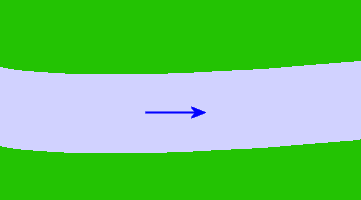I swim downstream at the rate of 1 m/s, as indicated by the dark red arrow below. (It is 4 times the length of the river current vector, indicating that my swimming velocity is 4 times the velocity of the river current.)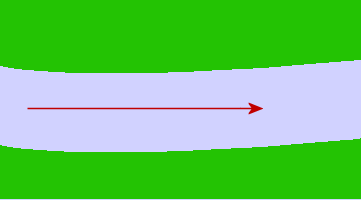Our friends are on the riverbank watching me swim. They observe that I am moving quite quickly. My velocity is:

1 + 0.25 = 1.25 m/s.

The river is helping me to move quickly relative to the people watching. This is an example of vector addition.

We can show this on our diagram as follows: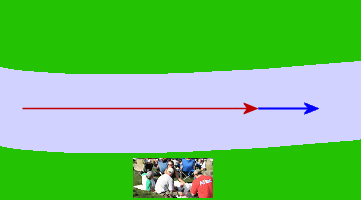The equivalent vector (1.25 m/s downstream) is shown in pink: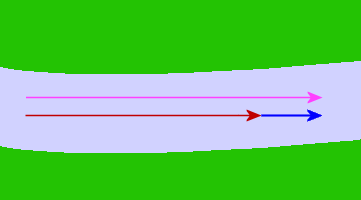The diagram illustrates the boost that I am getting from the river current and demonstrates my total speed, relative to the riverbank, of:

1 + 0.25 = 1.25 m/s.

The Return Journey (Subtraction of Vectors)

To get back to my friends, I need to swim against the current so my speed relative to the people who are watching me will be less. My velocity relative to the river bank is now:

1 − 0.25 = 0.75 m/s.

The 2 vectors are now acting in opposite directions.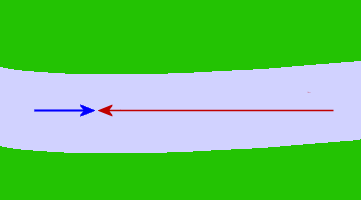An equivalent way of drawing our 2 vectors is to put the tail of the current vector next to the tip of the swimming vector as follows: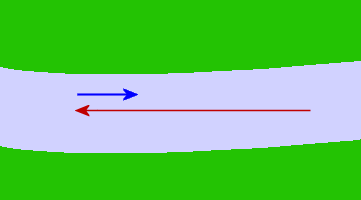My velocity relative to the people watching (0.75 m/s, upstream) is shown in pink: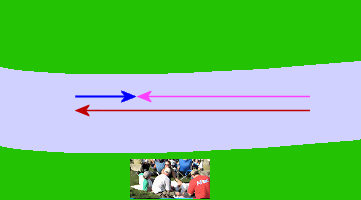## Problem SolverThis tool combines the power of mathematical computation engine that excels at solving mathematical formulas with the power of GPT large language models to parse and generate natural language. This creates math problem solver thats more accurate than ChatGPT, more flexible than a calculator, and faster answers than a human tutor. Learn More.